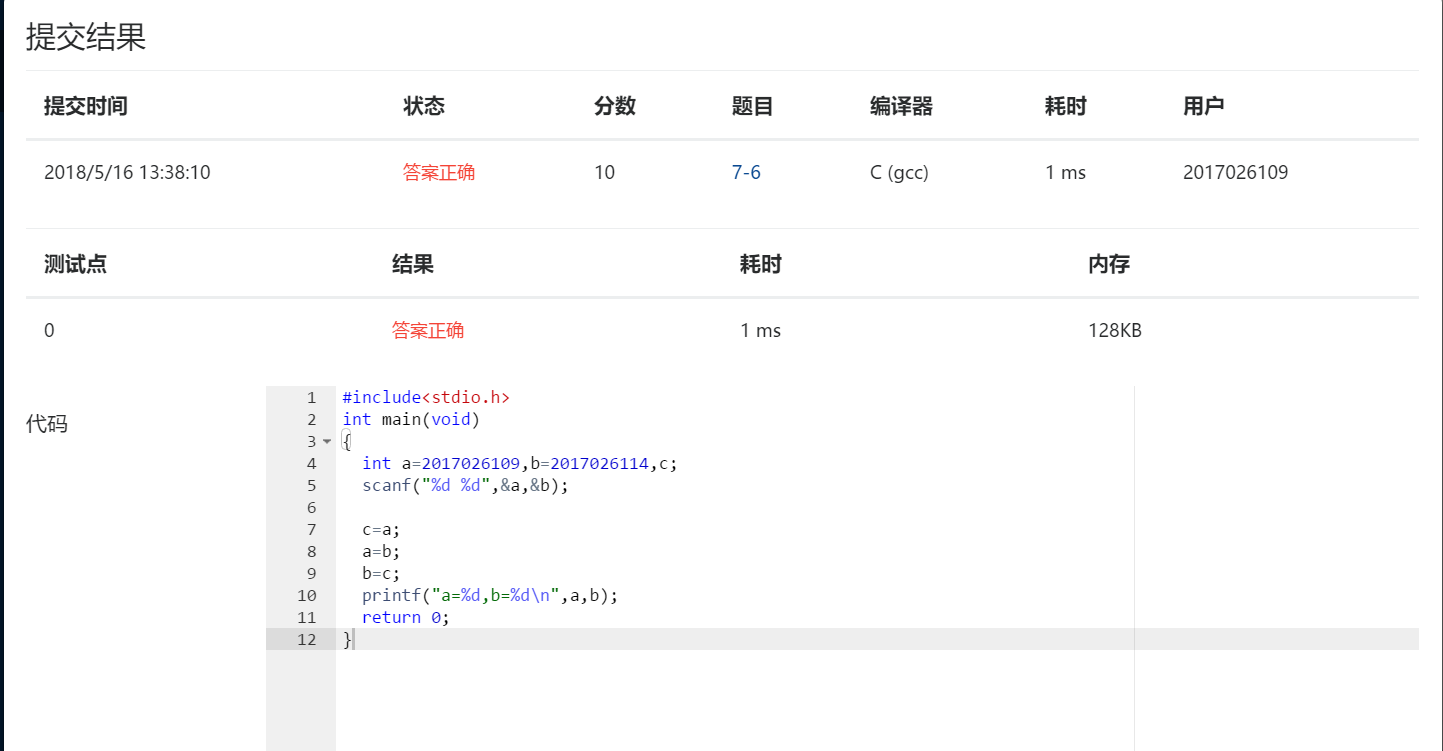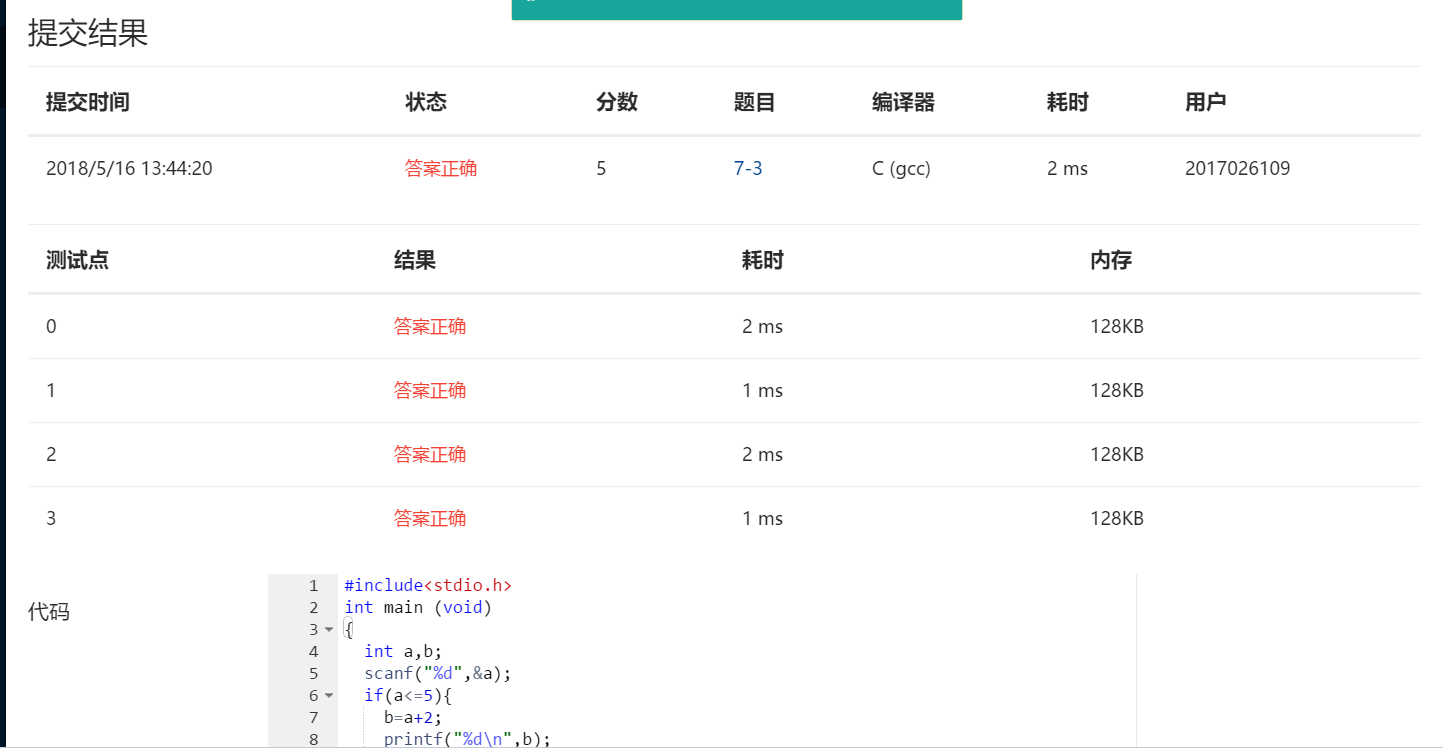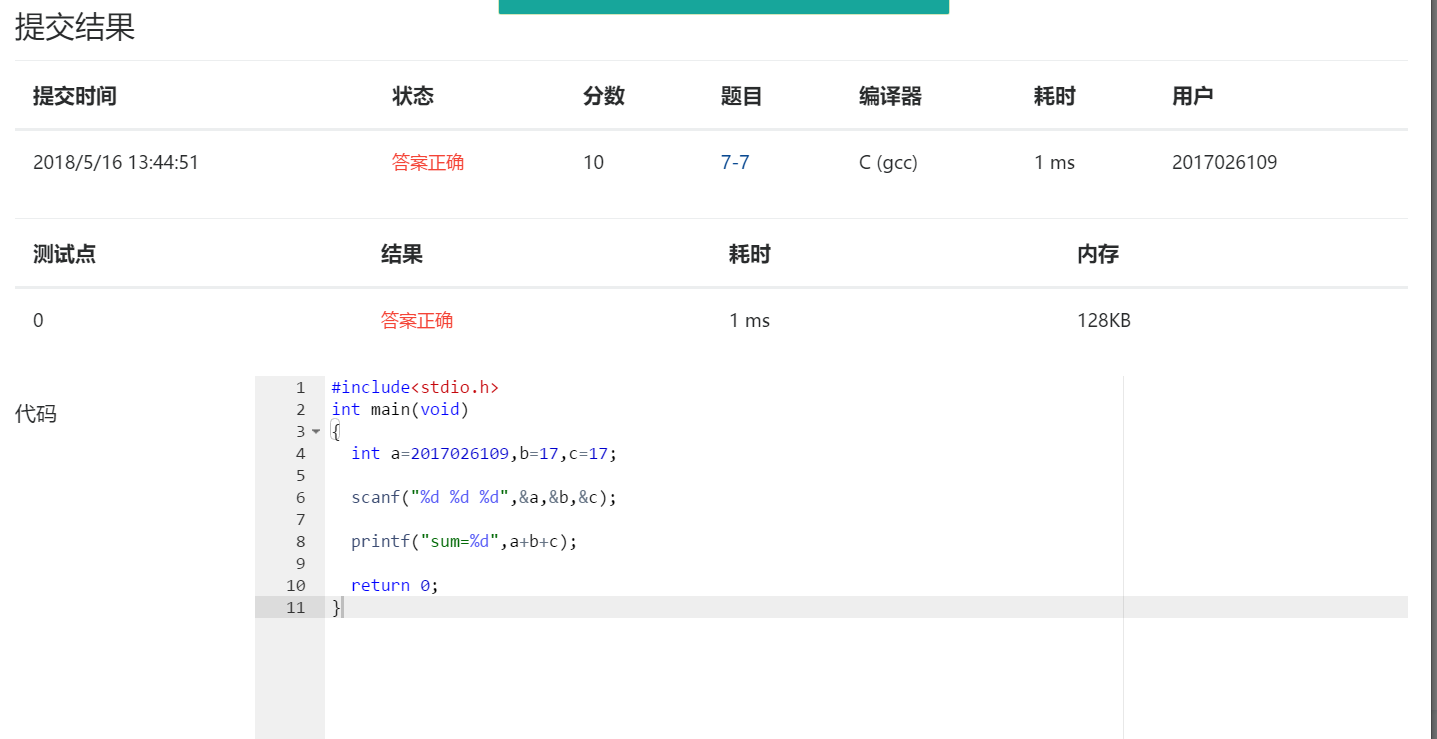# 第一次作业1.经过这几周的学习，总结一下学习的心得与体会。

• 问题1 ：循环语句如何使用？
• 问题1的解决方案：for（表达式1；表达式2；表达式3）
•                                       循环体语句
• 问题2 ：while语句如何使用？
• 问题2的解决方案：表达式1；
•                                while（表达式2）{
•                                    for的循环体语句；
•                                     表达式3；
•               }
• 问题3 浮点型怎么用？
• 问题3的解决方案：float（double）；

#include<stdio.h>
int main(void)
{
int a=2017026109,b=2017026114,c;
scanf("%d %d",&a,&b);

c=a;
a=b;
b=c;
printf("a=%d,b=%d\n",a,b);
return 0;
}2.

#include<stdio.h>
int main(void)
{
int a=2017026109,b=17,c=17;

scanf("%d %d %d",&a,&b,&c);

printf("sum=%d",a+b+c);

return 0;
}3.

#include<stdio.h>
int main (void)
{
int a,b;
scanf("%d",&a);
if(a<=5){
b=a+2;
printf("%d\n",b);
}
else{
b=a+2-7;
printf("%d\n",b);
}
return 0;
}MOOC

posted on 2018-05-03 21:59  香蕉你个不呐呐！  阅读(153)  评论(1编辑  收藏  举报